Question

# Three charges are placed on the y-axis. The first, with charge ﻿8nC, sits at y=5cm. The...

Three charges are placed on the y-axis. The first, with charge ﻿8nC, sits at y=5cm. The second, with charge -14nC, is at y=0cm. The third, -10﻿nC, is at y=−5cm.

a) What is the electric potential on the x-axis at x=10cm?

b) What is the magnitude of the electric field at x=10cm?

c) What is the electric potential at x= −10cm?

d) What is the magnitude of the electric field at x= −10cm?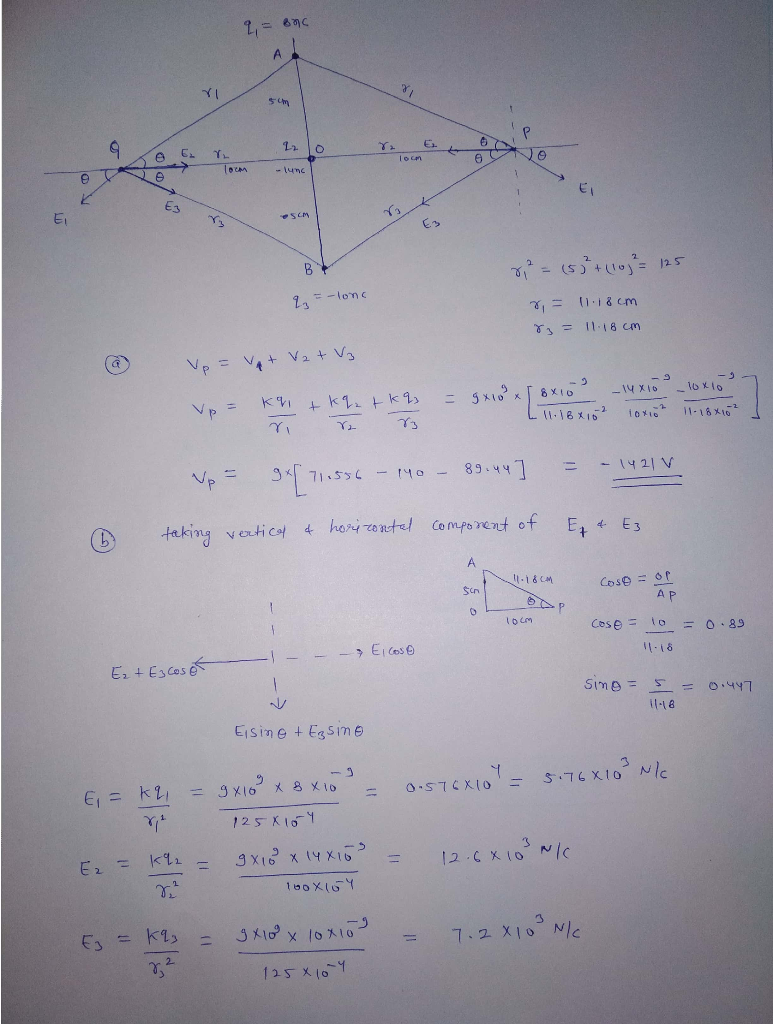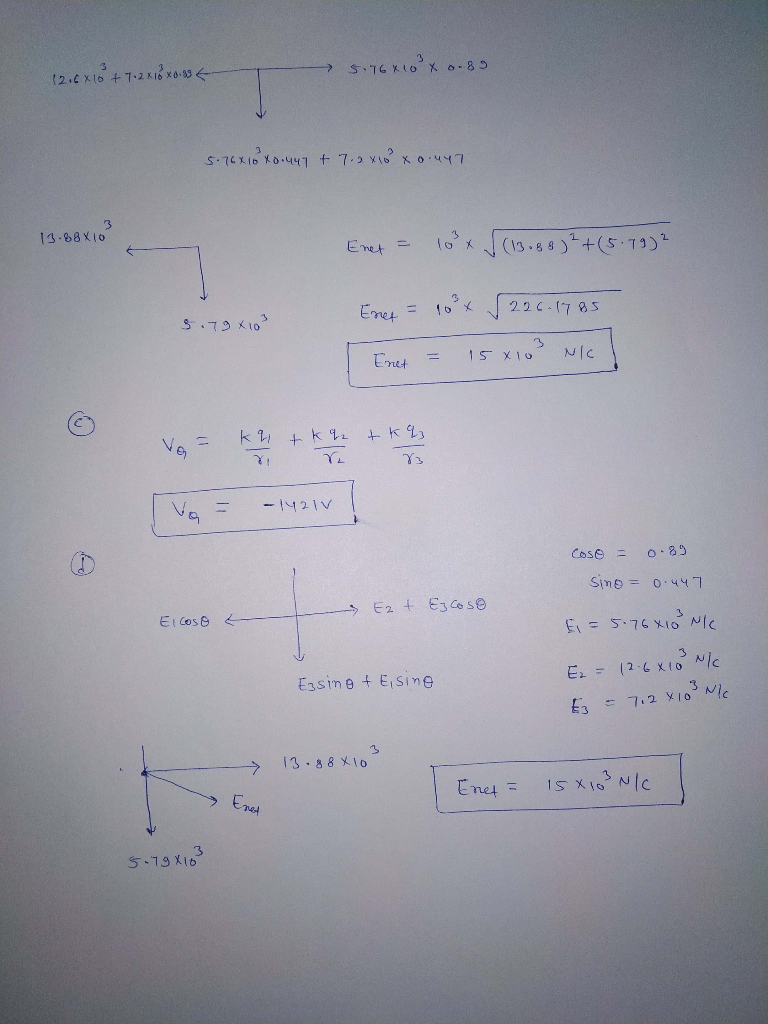#### Earn Coins

Coins can be redeemed for fabulous gifts.

Similar Homework Help Questions
• ### Three charges are placed on the y-axis. The first, with charge ﻿11nC, sits at y=5cm. The...

Three charges are placed on the y-axis. The first, with charge ﻿11nC, sits at y=5cm. The second, with charge -13nC, is at y=0cm. The third, -9﻿nC, is at y=−5cm. Part A What is the electric potential on the x-axis at x=10cm? Part B What is the magnitude of the electric field at x=10cm? Part C What is the electric potential at x=−10cm? Part D What is the magnitude of the electric field at x=−10cm?

• ### Two electrical charges q1 = 10nC and q2- -8nC are placed, the first charge being a sphere of radius "c" and the second loadinga spherical shell with internal radius "a" and exter...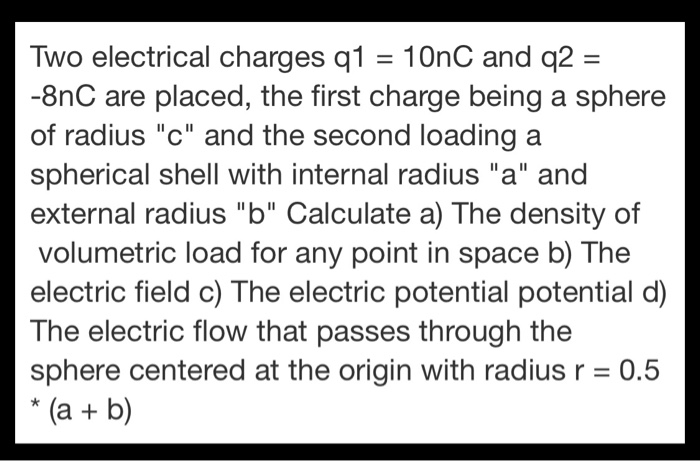Two electrical charges q1 = 10nC and q2- -8nC are placed, the first charge being a sphere of radius "c" and the second loadinga spherical shell with internal radius "a" and external radius "b" Calculate a) The density of volumetric load for any point in space b) The electric field c) The electric potential potential d) The electric flow that passes through the sphere centered at the origin with radius r 0.5 (a b) Two electrical charges q1 = 10nC...

• ### Three charges are located along y-axis with first -77 C charge at origin, second -100 C...

Three charges are located along y-axis with first -77 C charge at origin, second -100 C charge 2m above origin and third +50 C charge 3m below origin. (a) What is the magnitude and direction of force exerted by second charge on first charge, (b) what is the magnitude and direction of force exerted by third charge on first charge, (c) What is the net force on first charge? (d) If the third charge is replaced by an imaginary test...

• ### Three charges are located along y-axis with first -50 C charge at origin, second -100 C...

Three charges are located along y-axis with first -50 C charge at origin, second -100 C charge 2m above origin and third +50 C charge 3m below origin. (a) What is the magnitude and direction of force exerted by second charge on first charge, (b) what is the magnitude and direction of force exerted by third charge on first charge, (c) What is the net force on first charge? (d) If the third charge is replaced by an imaginary test...

• ### A positive charge (8nC) is located at y=0.2m on the y-axis, and a negative charge (-5nC)...

A positive charge (8nC) is located at y=0.2m on the y-axis, and a negative charge (-5nC) is located at x=0.7 on the x-axis. What is the electric potential at the origin as a result of these 2 charges?

• ### Three charges are configured as shown. A 3.0 nC charge is located at the origin. A -5.0 nC charge is located at a point on the y axis 0.1 m from the origin.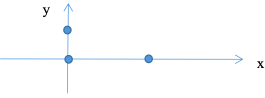Problem 1 Charge Configuration Three charges are configured as shown. A 3.0 nC charge is located at the origin. A -5.0 nC charge is located at a point on the y axis 0.1 m from the origin. The third charge of 4 nC is located on the x axis at a distance of 0.3 m from the origin. a) What is the magnitude and direction of the electric field at the origin? b) What is the magnitude and direction of the force on...

• ### 2. Two electrical charges on the x-axis, one charge of -3C at -1i and the second...

2. Two electrical charges on the x-axis, one charge of -3C at -1i and the second of 2C electrical charge at 1i. a) Calculate the electrical potential Φ for any point in xi and in particular for x = 2. b)Calculate the electric field generated by electric charges at any point xi and in particular at x = 2 c)At x = 2 a third electric charge with a mass m y of 1C is placed. What is the potential...

• ### Please show all work:) (a) Three point charges are placed along the y-axis, with charge ql...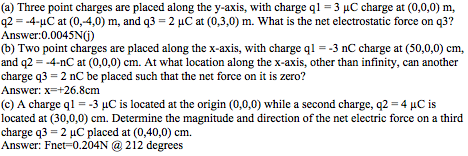Please show all work:) (a) Three point charges are placed along the y-axis, with charge ql -3 HC charge at (0,0,0) m, q2 --4-uC at (0,-4,0) m, and q3 -2 uC at (0,3,0) m. What is the net electrostatic force on q3? Answer:0.0045N0) (b) Two point charges are placed along the x-axis, with charge q 3 nC charge at (50,0,0) cm, and q2--4-nC at (0,0,0) cm. At what location along the x-axis, other than infinity, can another charge q3-2 nC...

• ### Two point charges are placed on the x axis. (Figure 1)The first charge, q1 = 8.00...

Two point charges are placed on the x axis. (Figure 1)The first charge, q1 = 8.00 nC , is placed a distance 16.0 m from the origin along the positive x axis; the second charge, q2 = 6.00 nC , is placed a distance 9.00 m from the origin along the negative x axis. Part A Part complete Calculate the electric field at point A, located at coordinates (0 m, 12.0 m ). Give the x and y components of...

• ### Three charges are placed on the z-axis. There is a charge of +7.90 pC placed at...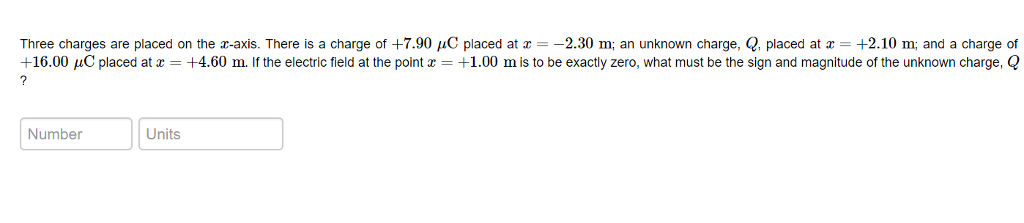Three charges are placed on the z-axis. There is a charge of +7.90 pC placed at z =-2.30 m, an unknown charge, Q, placed at x = +2.10 m; and a charge of +16.00 μC placed at x = +4.60 m. if the electric field at the point x = +1.00 m is to be exactly zero, what must be the sign and magnitude of the unknown charge, Q Number Units